you are here:/ News & Insights / Engineering Advantage Blog / What is Mass Scaling and When is it Appropriate in Explicit Dynamics Analysis?

# What is Mass Scaling and When is it Appropriate in Explicit Dynamics Analysis?October 17, 2014 By: Steven Hale

Have you ever painstakingly worked to develop an explicit dynamics model only to find out that it takes days to run?  Wouldn’t you like to be able to speed up your runs without sacrificing solution accuracy?  Mass scaling may provide a solution.

Run time for a transient dynamics model is not only a function of the model size, but also the time step size.  In explicit dynamics, the time step is internally calculated to provide a stable solution and to accurately predict the propagation of the highest frequency waves in the model; the stress and shock waves.  This condition limits the time step such that the stress wave cannot travel more than the smallest element dimension in a single step. This is known as the Courant condition which is shown in Equation 1.

Equation 1: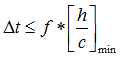Where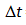= Stable time step

h = Smallest element dimension in the model

c = Acoustic wave speed (speed of stress wave)

f = Scale factor to improve stability (typically 0.9)

The equation for the acoustic wave speed is different for each element type but its simplest form is listed in Equation 2.

Equation 2: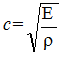Where E = Young’s modulus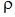= density

Request a free Explicit Dynamics Consulting Consultation today!

There are three parameters that can be adjusted to increase the time step and bring down the run time: element size, Young’s modulus, and density.  While the mesh can be adjusted to increase the element sizes, this is often impractical when geometries are complex with small features.  Young’s modulus is a material stiffness property and artificially lowering it will adversely affect accuracy.  Artificially increasing density can also adversely affect accuracy (think force = mass times acceleration).  But what if the density could be increased only in the smallest elements that control the time step? This idea is the basis for mass scaling.

Mass scaling is an automated procedure whereby the code increases the time step by scaling up the density in the specific elements that are controlling the time step.  The user specifies a minimum time step size and the density in those elements that have time steps smaller than this value are increased to the point where the time step is equal to this value.  While this is a very effective tool, it’s not without its drawbacks.

Mass scaling is a tried and proven method for reducing run times in quasi-static analyses where the velocity is low and the kinetic energy is very small relative to the internal energy (please refer to my previous blog post,“How Can Explicit Solvers Help with Stubborn Nonlinear Statics Model” for more information on quasi-static analyses).  But what about truly dynamic analyses where an accurate mass distribution is critical to the solution?

It is still possible to use mass scaling effectively for such models, but it should be done judiciously.  As a rule of thumb, mass can be added to non-critical regions of the model as long as it doesn’t significantly increase the overall mass of a part.  Validation requires checking both the relative increase in mass as well as the location of the added mass.  Only very small amounts of mass should be added in locations where critical results are being evaluated.

To demonstrate the effects of mass scaling, a model of a Polycarbonate ball with through holes impacting a rigid plate at 20 m/s was developed in ANSYS Workbench/LS-Dyna. The critical result used to predict damage in the ball is the maximum plastic strain in the through holes. Two different meshes were evaluated, as shown in Figure 1.  The image on the left shows the model with a default mesh and the image on the right shows the model with a fine mesh at the top.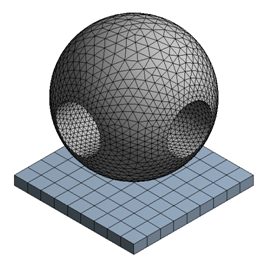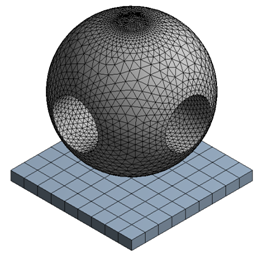Figure 1: Meshes – Polycarbonate Ball Impact Model

The two meshes were run with different levels of mass-scaling.  The plastic strain distribution for the default mesh without any mass scaling is shown in Figure 2.  All of the plastic strain occurs in the through holes.  We can safely assume, then, that scaling the mass in the small elements at the top of the ball in the second mesh should not affect the critical results because these elements are relatively far from the critical regions.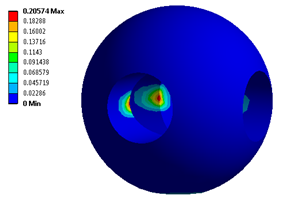Figure 2:  Plastic Strain Distribution – Default Mesh

A comparison of solutions for the two different meshes and different mass scaling values is provided in Table 1.  Notice that a small amount of mass scaling (dt = 3.e-7) assigned to the default mesh has a minimal impact on the plastic strain, while a larger amount of mass scaling (dt = 6.e-7) has a significant effect, even though it only causes in increase of 0.19% in the mass of the ball.  However, for the model with the fine mesh at the top, a significant increase in mass scaling (dt = 3.e-7 s up from 1.e-7 s) causes only a minor change in plastic strain while resulting in nearly a 60% drop in run time!  In this case, all of the mass scaling was applied to the smallest elements at the top of the ball, far from the through holes, as shown in Figure 3.

Request a free Explicit Dynamics Consulting Consultation today!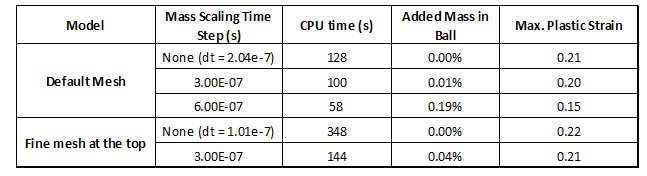Table 1:  Model Comparison Showing the Effects of Mass Scaling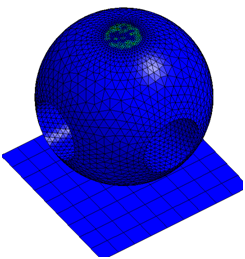Figure 3:  Added Mass (non-blue elements at top of ball)

In conclusion, mass scaling is a proven and effective tool for reducing run times in explicit dynamics analysis, especially in models that have complex geometry and small features that make mesh coarsening difficult.  While mass scaling is simple to apply, it needs to be done judiciously in models that are not quasi-static.  Analysts should check both the amount of mass scaling as a percentage of part mass and the location of the mass scaling to make sure that artificial mass is not being added to critical regions.  Small percentage increases in mass applied to critical locations can significantly impact the accuracy of critical results.  What good are results obtained quickly if they aren’t accurate?  It is always wise to run your model once without mass scaling and compare critical results to quantify the effects of mass scaling.

I am most interested in feedback from those of you that have used mass scaling in explicit dynamics analysis.  Have you found mass scaling to be a valuable tool for speeding up your runs without sacrificing accuracy?

Request a free Explicit Dynamics Consulting Consultation today!